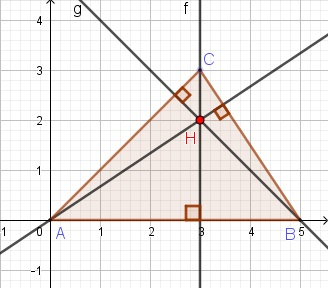# Altitudes, Medians and Angle Bisectors of a Triangle

The altitudes, medians and angle bisectors of a Triangle are defined and problems along with their solutions are presented.

## Altitudes of a Triangle

The altitude of a triangle is a line through a given vertex of the triangle and perpendicular to the side opposite to the vertex. For any triangle, all three altitudes intersect at a point called the orthocenter which may be inside or outside the triangle..
Example of triangle with othocenter inside the triangle.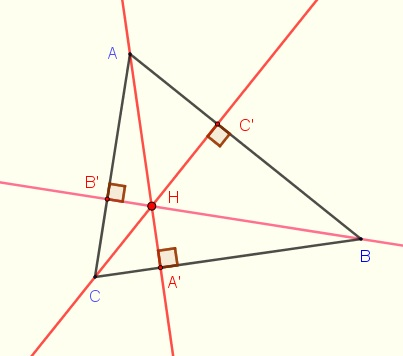Example of triangle with othocenter outside the triangle.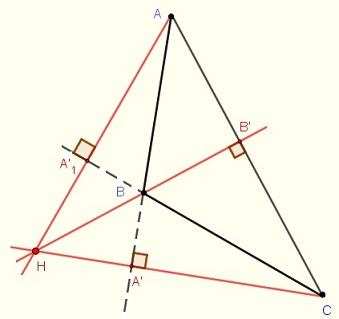## Medians of a Triangle

The median of a triangle is a line segment from a given vertex to the middle of the opposite side. For any triangle, all three altitudes intersect at a point called the centroid of the triangle.For a triangle with vertices
A(xA , yA), B(xB , yB) and C(xC , yC), then the centroid is at:
x = (x
A + xA + xA) / 3 , y = (yA + yB + yC) / 3
which are the averages of the x and y coordinates of the vertices.

## Angle Bisectors of a Triangle

The angle bisector of a triangle is a line segment from a given vertex that divide the interior angle at the vertex into two equal angles.If
A(xA , yA), B(xB , yB) and C(xC , yC) are the vertices of a triangle with sides of lengths a, b and c opposite vertices A, B and C respectively, then the incenter is at:
( (a x
A + b xB + c xC) / (a + b + c) , (a yA + b yB + c yC) / (a + b + c))

## Problems

Problem 1
Find the centroid of a triangle whose vertices are A(-2 , 0), B(4 , 3) and C(1 , 6).

Problem 2
Find the incenter of a triangle whose vertices are A(- 1 , 0), B(3 , 3) and C(3 , - 3).

Problem 3
Find the orthocenter of a triangle whose vertices are A(0 , 0), B(5 , 0) and C( 3 , 3)

## Solutions to the Above Problems

Solution to Problem 1
The x and y coordinate of the centroid are given by:
x = (- 2 + 4 + 1) / 3 = 1
y = (0 + 3 + 6) / 3 = 3
Below is shown the graphical solution including triangle, the medians of the three vertices and their point of intersection O called the centroid.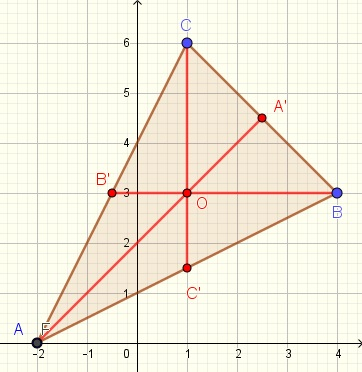Solution to Problem 2
The vertices are A(- 1 , 0), B(3 , 3) and C(3 , - 3).
We first calculate the lengths of the sides.
a = length of CB = √( (3 - 3) 2 + (3 - (-3)) 2 ) = 6
b = length of CA = √( (- 1 - 3) 2 + (0 - (-3)) 2 ) = 5
c = length of AB = √( (- 1 - 3) 2 + (0 - 3) 2 ) = 5
Use the formula given above to find the coordinate of the incenter
x = (a xA + b xB + c xC) / (a + b + c) = (6�(-1) + 5�3 + 5�3) / 16 = 24 / 16 = 6 / 4 = 3 / 2
y = (a yA + b yB + c yC) / (a + b + c) = (6�(0) + 5�3 + 5�(-3)) / 16 = 0
Below is shown the graphical solution including triangle, the angle bisectors of the three vertices and their point of intersection I called the incenter.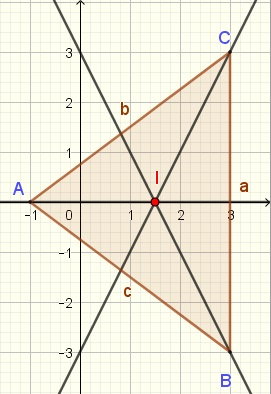Solution to Problem 3
The vertices are A(0 , 0), B(5 , 0) and C( 3 , 3).
The orthocenter is the intersection of all three altitudes from the vertices of the triangle.
We now need to find the equation of two altitudes and their point of intersection
1) The equation of the altitude through C is perpendicular to AB whose slope mC is given by
mC = (0 - 0) / (5 - 0) = 0
Therefore the line through A and B is a horizontal line. The altitude through C, which is perpendicular to AB, is a vertical line through C(3 , 3) and therefore its equation is x = 3.
2) The altitude through A is perpendicular to CB therefore the product of its slope mA and the slope mCB of the line through CB is equal to - 1.
Find slope mCB
mCB = (3 - 0)(3 - 5) = - 3 / 2
mCB � mA = - 1
Hence
mA = 2 / 3
The equation of the altitude through A(0 , 0) is given by
y = (2 / 3) x
The orthocenter is the point of intersection of the altitudes through O and C whose equations were found to be y = (2 / 3) x and x = 3 respectively.
The point of intersection is given by the solution to the system
y = (2 / 3) x and x = 3
whose solution is (3 , 2).

Below is shown the graphical solution including triangle, the altitudes of the three vertices and their point of intersection H called the orthocenter.